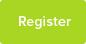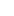# Tutor Hunt Questions

A 0.050 mol sample of a hydrocarbon was burned in excess oxygen.
The products were 3.60 g of water and 6.60 g of carbon dioxide.

Calculate the number of moles of carbon dioxide produced.
Relative atomic masses: C = 12 O = 16.

When the hydrocarbon was burned 0.20 mol of water were produced.
How many moles of hydrogen atoms are there in 0.20 mol of water?
3 years ago

Chemistry Question asked by Carley

6.6g of CO2 produced during the combustion, therefore RMM CO2 = 44 mol of CO2 produced = 6.6g/44g/mol = 0.15 mol Water formula = H20 0.2 mol of water produced and the RAM for H=1, RMM, H2O =18 so, 2/18=H/0.2 H=0.0222 mol
06/04/2016 18:08:06 | comment by Poh She
Know the Answer?

Please enter your response to the question below. The student will get a notification as soon your response has been approved by our moderation team.

## 2 Answers

We known that there is 0.05 mol of an unknown hydrocarbon we`ll call `X` and that it`s combustion forms 3.6 g of water and 6.6 g of carbon dioxide.

It would be sensible to convert these amounts to moles using:

amount (moles) = mass (grams) / relative atomic mass (g mol^-1)

therefore for H2O:
moles = 3.6 / 18 = 0.2

similarly for CO2:
moles = 6.6 / 44 = 0.15

now we can write the equation as:

0.05 X + x` O2 -> 0.15 CO2 + 0.2 H2O

this can be simplified by dividing through by 0.05

(this is probably the step that will be most useful to you)

giving:
1 X + x O2 -> 3 CO2 + 4 H2O

(try finishing from here before reading the rest)

____________________

All the carbon in the carbon dioxide and all of the hydrogen in the water must have come from the hydrocarbon.

This means 3 x 1 = 3 moles of C and 4 x 2 = 8 moles of H.

In a single molecule of X there are 3/1 = 3 Cs and 8/1 = 8 Hs

(Dividing by 1 seems pointless but it shows the procedure to follow if there were more than one mol of X in the initial equation)

This clearly gives C3H8 or propane as hydrocarbon X

Answered by Gregory | 3 years ago
CH4 + 2O² ==> 2H20 + CO2
(0.05m) (3.60g) (6.60g)
[hydrocarbon + excess oxygen]
No of moles of CO2 produced == mass/molarmass =6.60g/44g/mol
=0.15mole

[CO2(molarmass)= 12+16(2)=44g/mol]

(ii) moles of hydrogen in 0.2mole of H20
1mole of H2O contains 2moles of H
0.20mole of H2O will contain
0.2mole * 2moles = 0.4mole of Hydrogen
Answered by Zainab | 3 years agoPopular Links
© 2005-2019 Tutor Hunt - All Rights Reserved

Privacy Policy | Copyright | Terms of Service
loaded in 0.031 seconds